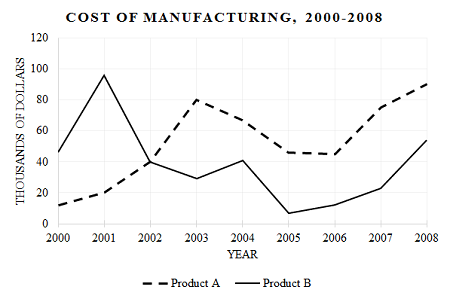# SAT Data - Graphs and ChartsThe graph above shows the cost of manufacturing of two products, A and B, per year. In which year was the difference in the cost of manufacturing the two products the greatest?

(A) $\ \ 2001$
(B) $\ \ 2002$
(C) $\ \ 2003$
(D) $\ \ 2007$
(E) $\ \ 2008$

×